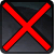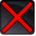# FR_StartcountdownConditions

## Related effects, buffs and debuffs

Please click on an effect below to view its details.

• [20s]FR_Startcountdown

 Slot: Buff Duration: 20s Tick rate: does not tick # occurrences: 0 Conditions: Can only by called by other effects
• On Apply

Perform the following actions:

• Custom
- Param 4 = (string) Warzone Begins in 30 Seconds
- Param 5 = (string)
- Param 6 = (string)
- Param 7 = (string)
- Param 8 = (string)
- Param 9 = (string)
- Param 1 = (int) 10199437207600779921
- Param 2 = (int) 7044208247444806032
- Param 3 = (int) 9955467716775914781
• When effect expires

Perform the following actions:

• Add effect #2 to TARGET from TARGET
• [5s]FR_Startcountdown

 Slot: Buff Duration: 5s Tick rate: does not tick # occurrences: 0 Conditions: Can only by called by other effects
• When effect is applied

Perform the following actions:

• Custom
- Param 4 = (string) Warzone Begins in 10 Seconds
- Param 5 = (string)
- Param 6 = (string)
- Param 7 = (string)
- Param 8 = (string)
- Param 9 = (string)
- Param 1 = (int) 10199437207600779921
- Param 2 = (int) 7044208247444806032
- Param 3 = (int) 9955467716775914781
• When effect expires

Perform the following actions:

• Add effect #3 to TARGET from TARGET
• [6s]FR_Startcountdown

 Slot: Buff Duration: 6s Tick rate: 1s Initial stacks: 5 Max stacks: 5 # occurrences: 0 Conditions: Can only by called by other effects
• When effect ticks

Perform the following actions:

• Custom
- Param 4 = (string) Warzone Begins in
- Param 5 = (string) Seconds
- Param 6 = (string)
- Param 7 = (string)
- Param 8 = (string)
- Param 9 = (string)
- Param 1 = (int) 10199437207600779921
- Param 2 = (int) 7044208247444806032
- Param 3 = (int) 935559496176630924
• Modify Charges
- Tag = (string)
- Ability Spec = (int) 0
- Amount Max = (float) -1
- Amount Min = (float) -1
- Amount Percent = (float) 0
• When effect expires

Perform the following actions:

• Custom
- Param 4 = (string) Bring Glory to your Faction!
- Param 5 = (string)
- Param 6 = (string)
- Param 7 = (string)
- Param 8 = (string)
- Param 9 = (string)
- Param 1 = (int) 10199437207600779921
- Param 2 = (int) 7044208247444806032
- Param 3 = (int) 9955467716775914781
• [30s]FR_Startcountdown

 Slot: Buff Duration: 30s Tick rate: does not tick # occurrences: 0
• When effect is applied

Perform the following actions:

• Custom
- Param 4 = (string) Warzone Begins in 60 Seconds
- Param 5 = (string)
- Param 6 = (string)
- Param 7 = (string)
- Param 8 = (string)
- Param 9 = (string)
- Param 1 = (int) 10199437207600779921
- Param 2 = (int) 7044208247444806032
- Param 3 = (int) 9955467716775914781
• When effect expires

Perform the following actions:

• Add effect #1 to TARGET from TARGET